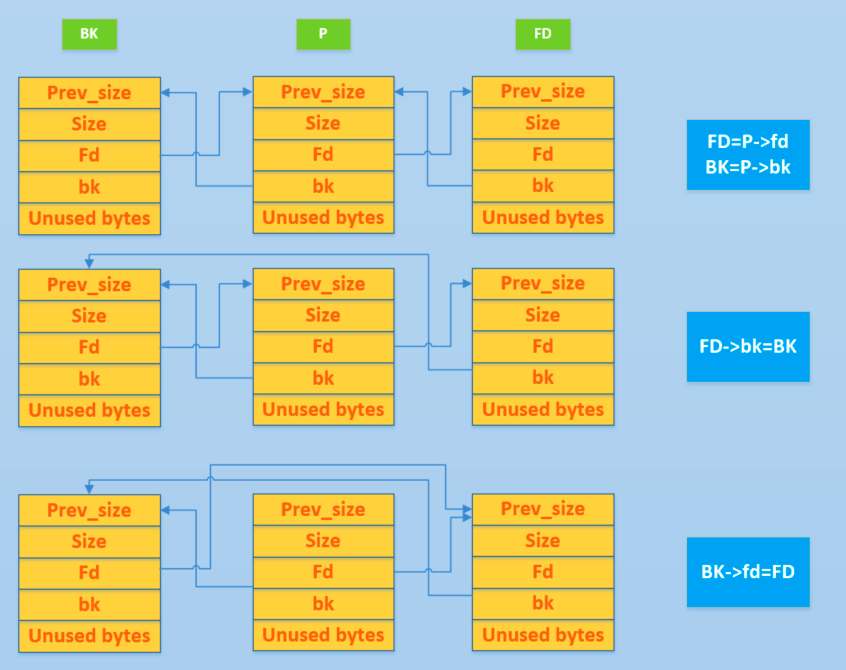# 基础操作¶

• malloc
• 从恰好大小合适的 large bin 中获取 chunk。
• 这里需要注意的是 fastbin 与 small bin 就没有使用 unlink，这就是为什么漏洞会经常出现在它们这里的原因。
• 依次遍历处理 unsorted bin 时也没有使用 unlink 。
• 从比请求的 chunk 所在的 bin 大的 bin 中取 chunk。
• free
• 后向合并，合并物理相邻低地址空闲 chunk。
• 前向合并，合并物理相邻高地址空闲 chunk（除了 top chunk）。
• malloc_consolidate
• 后向合并，合并物理相邻低地址空闲 chunk。
• 前向合并，合并物理相邻高地址空闲 chunk（除了 top chunk）。
• realloc
• 前向扩展，合并物理相邻高地址空闲 chunk（除了top chunk）。

/* Take a chunk off a bin list */
#define unlink(AV, P, BK, FD) {                                            \
// 由于 P 已经在双向链表中，所以有两个地方记录其大小，所以检查一下其大小是否一致。
if (__builtin_expect (chunksize(P) != prev_size (next_chunk(P)), 0))      \
malloc_printerr ("corrupted size vs. prev_size");               \
FD = P->fd;                                                                      \
BK = P->bk;                                                                      \
// 防止攻击者简单篡改空闲的 chunk 的 fd 与 bk 来实现任意写的效果。
if (__builtin_expect (FD->bk != P || BK->fd != P, 0))                      \
malloc_printerr (check_action, "corrupted double-linked list", P, AV);  \
else {                                                                      \
FD->bk = BK;                                                              \
BK->fd = FD;                                                              \
// 下面主要考虑 P 对应的 nextsize 双向链表的修改
// 如果P->fd_nextsize为 NULL，表明 P 未插入到 nextsize 链表中。
// 那么其实也就没有必要对 nextsize 字段进行修改了。
// 这里没有去判断 bk_nextsize 字段，可能会出问题。
&& __builtin_expect (P->fd_nextsize != NULL, 0)) {                      \
// 类似于小的 chunk 的检查思路
if (__builtin_expect (P->fd_nextsize->bk_nextsize != P, 0)              \
|| __builtin_expect (P->bk_nextsize->fd_nextsize != P, 0))    \
malloc_printerr (check_action,                                      \
"corrupted double-linked list (not small)",    \
P, AV);                                              \
// 这里说明 P 已经在 nextsize 链表中了。
// 如果 FD 没有在 nextsize 链表中
if (FD->fd_nextsize == NULL) {                                      \
// 如果 nextsize 串起来的双链表只有 P 本身，那就直接拿走 P
// 令 FD 为 nextsize 串起来的
if (P->fd_nextsize == P)                                      \
FD->fd_nextsize = FD->bk_nextsize = FD;                      \
else {                                                              \
// 否则我们需要将 FD 插入到 nextsize 形成的双链表中
FD->fd_nextsize = P->fd_nextsize;                              \
FD->bk_nextsize = P->bk_nextsize;                              \
P->fd_nextsize->bk_nextsize = FD;                              \
P->bk_nextsize->fd_nextsize = FD;                              \
}                                                              \
} else {                                                              \
// 如果在的话，直接拿走即可
P->fd_nextsize->bk_nextsize = P->bk_nextsize;                      \
P->bk_nextsize->fd_nextsize = P->fd_nextsize;                      \
}                                                                      \
}                                                                      \
}                                                                              \
}• libc 地址
• P 位于双向链表头部，bk 泄漏
• P 位于双向链表尾部，fd 泄漏
• 双向链表只包含一个空闲 chunk 时，P 位于双向链表中，fd 和 bk 均可以泄漏
• 泄漏堆地址，双向链表包含多个空闲 chunk
• P 位于双向链表头部，fd 泄漏
• P 位于双向链表中，fd 和 bk 均可以泄漏
• P 位于双向链表尾部，bk 泄漏

• 这里的头部指的是 bin 的 fd 指向的 chunk，即双向链表中最新加入的 chunk。
• 这里的尾部指的是 bin 的 bk 指向的 chunk，即双向链表中最先加入的 chunk。

// fd bk
if (__builtin_expect (FD->bk != P || BK->fd != P, 0))                      \
malloc_printerr (check_action, "corrupted double-linked list", P, AV);  \

// next_size related
if (__builtin_expect (P->fd_nextsize->bk_nextsize != P, 0)              \
|| __builtin_expect (P->bk_nextsize->fd_nextsize != P, 0))    \
malloc_printerr (check_action,                                      \
"corrupted double-linked list (not small)",    \
P, AV);


## malloc_printerr¶

static void malloc_printerr(const char *str) {
__libc_message(do_abort, "%s\n", str);
__builtin_unreachable();
}


  if ((action & do_abort)) {
if ((action & do_backtrace))
BEFORE_ABORT(do_abort, written, fd);

/* Kill the application.  */
abort();
}


abort 函数里，在 glibc 还是2.23 版本时，会 fflush stream。

  /* Flush all streams.  We cannot close them now because the user
might have registered a handler for SIGABRT.  */
if (stage == 1)
{
++stage;
fflush (NULL);
}